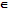# What is a normalizer or centralizer

Publish On: 2019-03-16

Total Post: 542

# Mjay Jollay

Total Post: 0

## ANS: What is a normalizer or centralizer

The set of all those elements of G, which commute with a given element a is called the normalizer of a and will be denoted by N (a).

Thus, N (a) = {xG : ax = xa}.

We have the following results.

(i) Since, ex = xe ∀ xG ; so N (e) = G.

i.e. the normalizer of the identity element is the whole group G.

(ii) if aG and G is abelian, then ax = xa, ∀ xG.

Consequently, N (a) = G.

i.e. the normalizer of every element of an abelian group G is always a sub-group of G.